Courses

# Test: Circuit Theorems- 2

## 10 Questions MCQ Test Topicwise Question Bank for Electrical Engineering | Test: Circuit Theorems- 2

Description
This mock test of Test: Circuit Theorems- 2 for Electrical Engineering (EE) helps you for every Electrical Engineering (EE) entrance exam. This contains 10 Multiple Choice Questions for Electrical Engineering (EE) Test: Circuit Theorems- 2 (mcq) to study with solutions a complete question bank. The solved questions answers in this Test: Circuit Theorems- 2 quiz give you a good mix of easy questions and tough questions. Electrical Engineering (EE) students definitely take this Test: Circuit Theorems- 2 exercise for a better result in the exam. You can find other Test: Circuit Theorems- 2 extra questions, long questions & short questions for Electrical Engineering (EE) on EduRev as well by searching above.
QUESTION: 1

Solution:
QUESTION: 2

Solution:
QUESTION: 3

### Match List- I (Theorem/Law) with List- II (Property) and select the correct answer using the codes given below the lists: List - I A. Norton’s theorem B. Superposition theorem C. Thevenin’s theorem D. Kirchhoff’s current law List - II 1. Effects of independent sources in a linear circuit are additive. 2. Law of non-accumulation of charge holds good at nodes. 3. Current source with shunt resistor. 4. Voltage source with series resistor. Codes: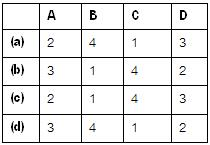Solution:
QUESTION: 4

The v-i characteristic of an element is shown in figure below. The element is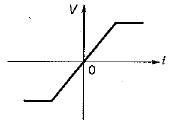Solution:
QUESTION: 5

If the source impedance is inductive, for maximum transfer of power from the source to the load, the load should be

Solution:
QUESTION: 6

The equivalent resistance between terminal A and B for the given circuit is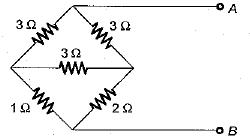Solution:

Converting 3 Ω delta connected resistors into star-equivalent, the circuit is reduced as shown below.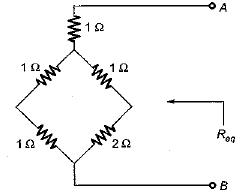∴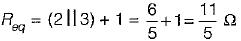QUESTION: 7

Assertion (A): Norton’s theorem is called dual of the Thevenin’s theorem.
Reason (R): If the Thevenin’s equivalent voltage source is converted to an equivalent current source, the Norton’s equivalent is obtained.

Solution:
QUESTION: 8

Assertion (A): In any active network, maximum power transfer to the load takes place when the load impedance is equal to an equivalent impedance of the network as viewed from the terminals of the load.
Reason (R): For finding the maximum power transfer to the load, any network has to be first converted into Th even in’s equivalent across the terminals of the load.

Solution:

ZL = Z*S for maximum power transfer. Hence, assertion is a false statement.

QUESTION: 9

Assertion (A): The substitution theorem is very useful in the networks consisting the mutual inductances.
Reason (R): By the substitution theorem, the effect of mutual inductances may be replaced by equivalent dependent sources of the same Value.

Solution:
QUESTION: 10

Consider the following statements related to
Thevenin’s theorem:
For the application of Thevenin’s theorem
1. the circuit should not. contain non-iinear elements.
2. the circuit may contain unilateral or bilateral elements or both.
3. there should not be magnetic coupling between the load and circuit, to be replaced by Thevenin's theorem.
4. there should not be controlled sources on the load size, controlled from some other oart of the circuit.

Solution: